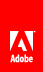# Using Rectangle objects

Flash Player 9 and later, Adobe AIR 1.0 and later

A Rectangle object defines a rectangular area. A Rectangle object has a position, defined by the x and y coordinates of its upper-left corner, a width property, and a height property. You can define these properties for a new Rectangle object by calling the Rectangle() constructor function, as follows:

```import flash.geom.Rectangle;
var rx:Number = 0;
var ry:Number = 0;
var rwidth:Number = 100;
var rheight:Number = 50;
var rect1:Rectangle = new Rectangle(rx, ry, rwidth, rheight);```

## Resizing and repositioning Rectangle objects

There are a number of ways to resize and reposition Rectangle objects.

You can directly reposition the Rectangle object by changing its x and y properties. This change has no effect on the width or height of the Rectangle object.

```import flash.geom.Rectangle;
var x1:Number = 0;
var y1:Number = 0;
var width1:Number = 100;
var height1:Number = 50;
var rect1:Rectangle = new Rectangle(x1, y1, width1, height1);
trace(rect1) // (x=0, y=0, w=100, h=50)
rect1.x = 20;
rect1.y = 30;
trace(rect1); // (x=20, y=30, w=100, h=50)```

As the following code shows, when you change the left or top property of a Rectangle object, the rectangle is repositioned. The rectangle’s x and y properties match the left and top properties, respectively. However, the position of the lower-left corner of the Rectangle object does not change, so it is resized.

```import flash.geom.Rectangle;
var x1:Number = 0;
var y1:Number = 0;
var width1:Number = 100;
var height1:Number = 50;
var rect1:Rectangle = new Rectangle(x1, y1, width1, height1);
trace(rect1) // (x=0, y=0, w=100, h=50)
rect1.left = 20;
rect1.top = 30;
trace(rect1); // (x=20, y=30, w=80, h=20)```

Similarly, as the following example shows, if you change the bottom or right property of a Rectangle object, the position of its upper-left corner does not change. The rectangle is resized accordingly:

```import flash.geom.Rectangle;
var x1:Number = 0;
var y1:Number = 0;
var width1:Number = 100;
var height1:Number = 50;
var rect1:Rectangle = new Rectangle(x1, y1, width1, height1);
trace(rect1) // (x=0, y=0, w=100, h=50)
rect1.right = 60;
trect1.bottom = 20;
trace(rect1); // (x=0, y=0, w=60, h=20)```

You can also reposition a Rectangle object by using the offset() method, as follows:

```import flash.geom.Rectangle;
var x1:Number = 0;
var y1:Number = 0;
var width1:Number = 100;
var height1:Number = 50;
var rect1:Rectangle = new Rectangle(x1, y1, width1, height1);
trace(rect1) // (x=0, y=0, w=100, h=50)
rect1.offset(20, 30);
trace(rect1); // (x=20, y=30, w=100, h=50)```

The offsetPt() method works similarly, except that it takes a Point object as its parameter, rather than x and y offset values.

You can also resize a Rectangle object by using the inflate() method, which includes two parameters, dx and dy . The dx parameter represents the number of pixels that the left and right sides of the rectangle moves from the center. The dy parameter represents the number of pixels that the top and bottom of the rectangle moves from the center:

```import flash.geom.Rectangle;
var x1:Number = 0;
var y1:Number = 0;
var width1:Number = 100;
var height1:Number = 50;
var rect1:Rectangle = new Rectangle(x1, y1, width1, height1);
trace(rect1) // (x=0, y=0, w=100, h=50)
rect1.inflate(6,4);
trace(rect1); // (x=-6, y=-4, w=112, h=58)```

The inflatePt() method works similarly, except that it takes a Point object as its parameter, rather than dx and dy values.

## Finding unions and intersections of Rectangle objects

You use the union() method to find the rectangular region formed by the boundaries of two rectangles:

```import flash.display.*;
import flash.geom.Rectangle;
var rect1:Rectangle = new Rectangle(0, 0, 100, 100);
trace(rect1); // (x=0, y=0, w=100, h=100)
var rect2:Rectangle = new Rectangle(120, 60, 100, 100);
trace(rect2); // (x=120, y=60, w=100, h=100)
trace(rect1.union(rect2)); // (x=0, y=0, w=220, h=160)```

You use the intersection() method to find the rectangular region formed by the overlapping region of two rectangles:

```import flash.display.*;
import flash.geom.Rectangle;
var rect1:Rectangle = new Rectangle(0, 0, 100, 100);
trace(rect1); // (x=0, y=0, w=100, h=100)
var rect2:Rectangle = new Rectangle(80, 60, 100, 100);
trace(rect2); // (x=120, y=60, w=100, h=100)
trace(rect1.intersection(rect2)); // (x=80, y=60, w=20, h=40)```

You use the intersects() method to find out whether two rectangles intersect. You can also use the intersects() method to find out whether a display object is in a certain region of the Stage. For the following code example, assume the coordinate space of the display object container that contains the circle object is the same as that of the Stage. The example shows how to use the intersects() method to determine if a display object, circle , intersects specified regions of the Stage, defined by the target1 and target2 Rectangle objects:

```import flash.display.*;
import flash.geom.Rectangle;
var circle:Shape = new Shape();
circle.graphics.lineStyle(2, 0xFF0000);
circle.graphics.drawCircle(250, 250, 100);
var circleBounds:Rectangle = circle.getBounds(stage);
var target1:Rectangle = new Rectangle(0, 0, 100, 100);
trace(circleBounds.intersects(target1)); // false
var target2:Rectangle = new Rectangle(0, 0, 300, 300);
trace(circleBounds.intersects(target2)); // true```

Similarly, you can use the intersects() method to find out whether the bounding rectangles of two display objects overlap. Use the getRect() method of the DisplayObject class to include any additional space that the strokes of a display object add to a bounding region.

## Other uses of Rectangle objects

Rectangle objects are used in the following methods and properties:

Class

Methods or properties

Description

BitmapData

applyFilter(), colorTransform(), copyChannel(), copyPixels(), draw(), drawWithQuality(), encode(), fillRect(), generateFilterRect(), getColorBoundsRect(), getPixels(), merge(), paletteMap(), pixelDissolve(), setPixels(), and threshold()

Used as the type for some parameters to define a region of the BitmapData object.

DisplayObject

getBounds(), getRect(), scrollRect, scale9Grid

Used as the data type for the property or the data type returned.

PrintJob

Used to define the printArea parameter.

Sprite

startDrag()

Used to define the bounds parameter.

TextField

getCharBoundaries()

Used as the return value type.

Transform

pixelBounds

Used as the data type.// Ethnio survey code removed